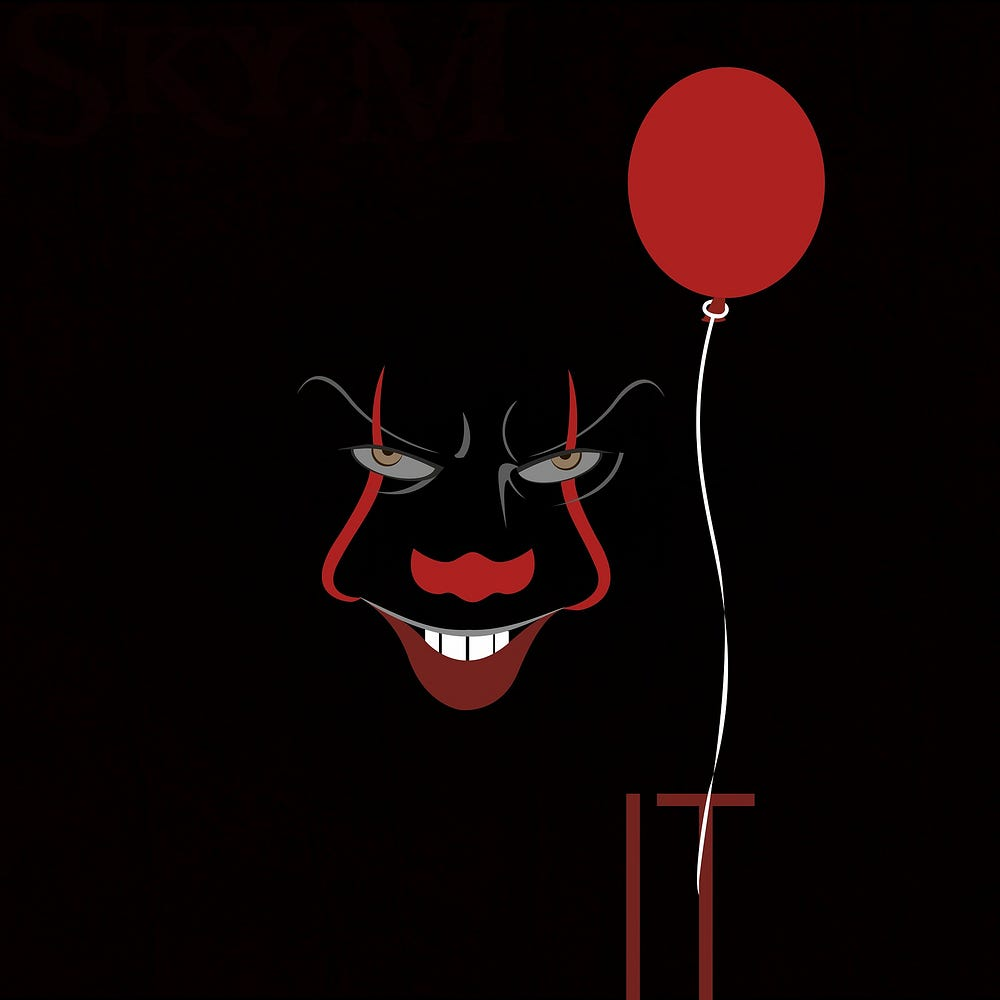# CNN approach for predicting Movie Genre from Posters!

Original article was published on Deep Learning on Medium# CNN approach for predicting Movie Genre from Posters!

Convolutional Neural Network is a class of deep neural networks and is used for image or video recognition and classification tasks.

Can we build a model that can learn what makes a movie poster of one genre different from others? The movie poster depicts many things about the movie. It plays a vital role in exciting the viewer’s interest in the movie. In above example, the colors are mainly red and black so when training our model may learn to classify this type of images as ‘horror’ or ‘thriller’. This will be an interesting task to do. When you look at the posters of different genres you notice that they are different in some ways. For example, look at the poster below. All images of movie posters used in this article are collected from the IMDB.

It represents a comedy movie. Now take a look at the poster below that is of an action movie. We can see that posters represent an important aspect of the movie genre.

In this project, we will build a neural model that can distinguish between three movie genre posters and predict any random poster’s genre. We will build this model step by step from scratch! The dataset used in this project is self-created with the help of IMDB. It contains over 3900 images of posters of each genre — Action, Comedy, Drama.

Let’s get to the coding portion.

# 1. Working with the dataset

Our dataset is structured as shown below. We have kept training images and test images in different directories. Each directory contains three subdirectories — Action, Comedy, and Drama. These subdirectories further contain the images of our movie posters.

We will use ImageDataGenerator for labeling purposes. ImageDataGenerator from Keras API helps us by labeling the data automatically. It is also useful when implementing data augmentation in your code. Let’s see how this is done in coding.

`import tensorflow as tfimport keras_preprocessingfrom keras_preprocessing import imagefrom keras_preprocessing.image import ImageDataGeneratorTRAINING_DIR = "/images2/Training"training_datagen = ImageDataGenerator(rescale = 1./255, width_shift_range=0.2, height_shift_range=0.2, zoom_range=0.2, horizontal_flip=True, fill_mode='nearest')VALIDATION_DIR = "/images2/Validation"validation_datagen = ImageDataGenerator(rescale = 1./255)train_generator = training_datagen.flow_from_directory( TRAINING_DIR, target_size=(150,150), class_mode='categorical', batch_size = 256)validation_generator = validation_datagen.flow_from_directory( VALIDATION_DIR, target_size=(150,150), class_mode='categorical', batch_size= 64)`

We will first create an instance of ImageDataGenerator for both training and validation purposes. As pixel values range from 0 to 255 we will normalize them in range 0 to 1. To do this we will pass in the argument (rescale = 1./255) when creating an instance of ImageDataGenerator. After this, we will use the .flow_from_directory() method of the instance to label the images for both directories and store the result in train_generator and validation_generator for training and validation purposes. While calling this method we will pass in the target_size attribute to ensure that our images in the dataset are of the same size. Here we have 3 classes so we have to pass in class_mode parameter as categorical. Batch sizes for both training and validation depend on the number of images we have in our dataset.

We have labeled our data into three classes — Action, Comedy, Drama. Now we can go ahead and create our CNN model.

# 2. Creating the CNN model

We will use Keras’ sequential model for building our model. We will add 3 pairs of Conv2D and MaxPooling2D layers. Then we will add the Flatten layer so that we have our data in one dimension. Finally, we will add a fully connected Dense layer with 1024 hidden units followed by a softmax layer. Below is the code to implement this.

`from tensorflow.keras.optimizers import RMSpropmodel = tf.keras.models.Sequential([ tf.keras.layers.Conv2D(16, (3,3), activation='relu', input_shape=(150, 150, 3)), tf.keras.layers.MaxPooling2D(2,2), tf.keras.layers.Conv2D(32, (3,3), activation='relu'), tf.keras.layers.MaxPooling2D(2,2), tf.keras.layers.Conv2D(64, (3,3), activation='relu'), tf.keras.layers.MaxPooling2D(2,2), tf.keras.layers.Flatten(), tf.keras.layers.Dense(1024, activation='relu'), tf.keras.layers.Dense(3, activation='softmax')])model.compile(loss='categorical_crossentropy', optimizer=RMSprop(lr=0.001), metrics=['acc'])`

After we create our model we will use the RMSprop optimizer when compiling the model which allows us to tweak the learning rate as needed. Here, the learning rate is chosen after many tests with the model. We will need to pass categorical_crossentropy as our loss function as we have more than two classes.

Our model is ready for training. Let’s train it with the data we labeled earlier.

# 3. Training the model

We will pass in the train_generator and validation_generator variables we created earlier with the right values for epochs.

`history = model.fit( train_generator, steps_per_epoch = 36, epochs = 100, validation_data = validation_generator, validation_steps = 36)`

After 100 epochs our model gave 69.8% training accuracy while validation accuracy was still 53.3%. Below is the chart plotted for both accuracy metrics.